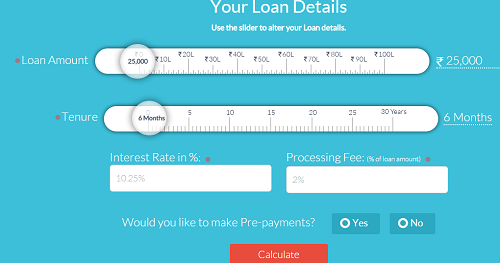# How to calculate emi plz help

08 November 2013

HOW TO CALCULATE LOAN EMI WITH INTERST

PRINCIPAL 100000/-

RATE 10%

DURATION 15 YEARS

KINDLY SOLVE WITH EASY DETAIL

Sandeep Garg (Consolidation and IndAS Application)   (1056 Points)
Replied 08 November 2013

For the given parameters EMI for 15 years would be Rs. 1074.61

Same can be calculated by using PPMT and IPMT formula in excel

Thanks

CA Suraj Lakhotia (IndigoLearn) (4898 Points)
Replied 08 November 2013

you can also use www.emicalculator.net

Replied 08 November 2013

but sir , let me know how solve this ?

Sandeep Garg (Consolidation and IndAS Application)   (1056 Points)
Replied 08 November 2013

EMI calculator attached for your reference.

Replied 08 November 2013

thanx sir but i want to solve this practicaly

Replied 08 November 2013

without any formula in excel or without any software

Krishna Teja (Chartered Accountant) (1007 Points)
Replied 08 November 2013

EMI= Principal / Present value annuity Factor (r,n)

so here EMI=100000 / PVAF(10%,15y)=100000/7.6061=Rs 13,147

So emi is Rs 13147

Siddhartha (Student Audit Assistant)   (272 Points)
Replied 08 November 2013

 Originally posted by : krishna tejaEMI= Principal / Present value annuity Factor (r,n)   so here EMI=100000 / PVAF(10%,15y)=100000/7.6061=Rs 13,147   So emi is Rs 13147The amount calculated above is called Equated Annual Installment (EAI) not Equated monthly Installment (EMI)

Deepak Gupta (CA Student) (15852 Points)
Replied 08 November 2013

CALCULATING EQUATED MONTHLY INSTALLMENT (EMI)
.
In house finance, equated monthly installment (EMI) refers to the monthly payment towards interest and principal made by a borrower to a lender. EMI is calulated using a formula that considers loan amount, interest rate, and loan period as variables.
.
The formula for calculating EMI:
EMI = (L × I) × [(1 + I)^N  ÷ {(1 + I)^N } -1]
.
Where
L = loan amount
l = interest rate per annum divided by 12
^ = to the power of
N = loan period in months
.
Assuming a loan of Rs. 1 lakh at 9 % per annum, repayable in 15 years, the EMI calculation using the formula will be:
EMI = (1,00,000 × 0.0075) × [(1 + 0.0075) 180  ÷ {(1+0.0075) 180} - 1]
= 750 × [3.838 ÷ 2.838]
= 750 × 1.35236
= 1,014

SARAVANAN.V (Legal Advisor ) (27 Points)
Replied 11 November 2013

Nice answer from Depak gupta and teja Reply To Others... As a professional or professional student don't give general answer like excel formula, website help... etc... பிரியாணி எப்படி செய்யறதுனு கேட்டா ஓட்டலில் கிடைக்கும்னு சொல்லாதீங்க

Replied 11 November 2013

This is the formula to caluculate: E = P×r×(1 + r)n/((1 + r)n - 1). E is EMI

RUSHIRAJSINH UMEDSINH JADEJA (N.A) (28 Points)
Replied 11 November 2013

Sir I want to know why interest rate divided by 1200 in excel calculator

Sandeep Garg (Consolidation and IndAS Application)   (1056 Points)
Replied 11 November 2013

this is 12 X 100

Since EMI is monthly so annual rate is divided by 12

Shruthi (Student Others) (25 Points)
Replied 04 June 2015

The Mathematical formula for calculating EMI = [P x R x (1+R) ^n] / [(1+R)^ n-1].

P is the principal loan amount,

R rate of interest per month and

N is the the number of monthly instalments.

Manual calculations are too complicated to perform accurately, which is why many borrowers are left confused after availing a loan. Understanding this pain-point led BankBazaar to develop one of the easiest and most user-friendly online Loan EMI Calculators.

I saw one of the best website here you just enter Loan amount, Tenure and Interest rate you will get how much you will monthly EMI.

Source: https://www.bankbazaar.com/finance-tools/emi-calculator.H T M Lview more »

﻿